# blog posts# Correlation tests are a process of measuring the extent to which two metric (standard) variables are related in some populations.

The amount that is usually denoted by a number is called the correlation coefficient.

In the continuation of spss software application tutorials , in this post, we will introduce you to the Pearson correlation test in SPSS.

## 2- Pearson correlation test training article

If you did not learn the correlation test through the video above, read this article. There are a number of correlation coefficients, but correlation usually means producing the torque correlation coefficient, which is called Pearson correlation (unless otherwise explained). Be. Hypothesis zero indicates that there is no linear relationship between the variables, which is called the zero correlation coefficient. The following figure illustrates this basic idea.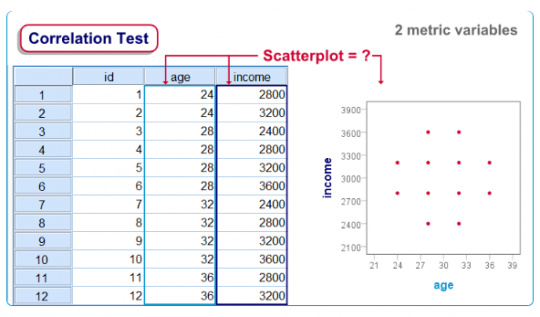## SPSS Correlation Test Example

A politician wants to know if age and net monthly income are related? He asks 30 respondents the question: Are the results obtained by age-gender in the sav file? Does this data determine the probability that age and income in the study population are related? (Combination) synax opens the following data: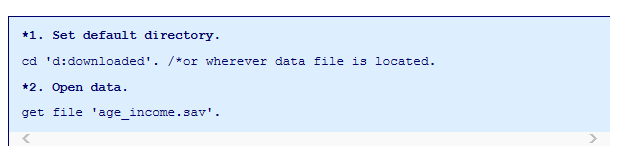## Quick Data Check

Before performing any statistical test, we first want to have an idea of ​​what the data is basically like. A good option here is the scatter plot. Screenshots get you started.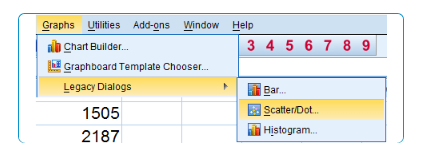• First we go to graphs> legacy dilaogs> scatter / dot .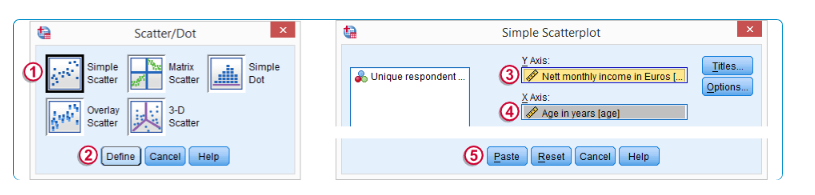• Then select the simple scatter .
• Click on Define .
• We transfer the revenue to the Y axis.
• Age to the X axis.
• Put the results in the following combination:

## Resulting Scatter Plot Results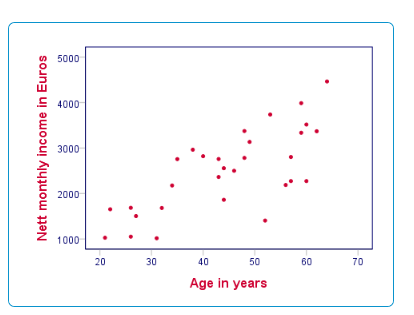In this case, the scatter plot shows that all respondents are between 20 and 68 years old. Ages are logically dispersed by an average of 45 years. Then the amount of income is from 1000 Euros to 4500 Euros. This is the kind of domain we expect for a monthly income in a developed country. In addition to how age and income are distributed separately, we also see that older respondents tend to have higher incomes. This indicates a positive correlation between age and income.

## Assumptions Correlation Test Assumptions

Interpreting the correlation coefficient alone does not require any assumptions. Although an important test for a correlation creates some assumptions. Independent observations (or even uniformly distributed and independent variables up to and more precisely). The sample size is logically large (N> 30)

## Run SPSS Correlation Test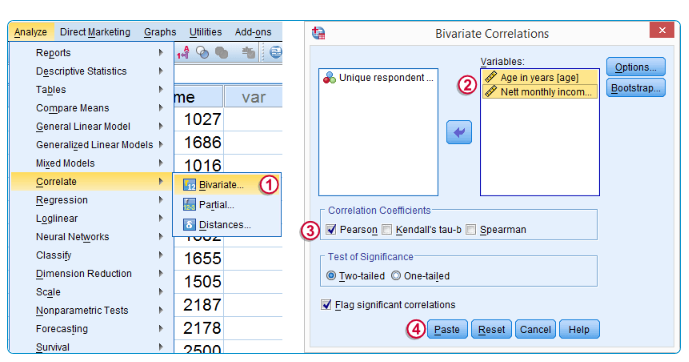The screenshots above show a standard way to get correlations, because we do them differently. This output data and combinations become cluttered. We can only type and execute the age-gender correlation. We think this is a faster and cleaner way to get a perfect correlation matrix. Note that we can use the keywords all and to in these commands. If we have multiple variables, a better suggestion is to conclude with a more regular output using the with keyword shown in the combination, as you know this regular output cannot be obtained from the menu.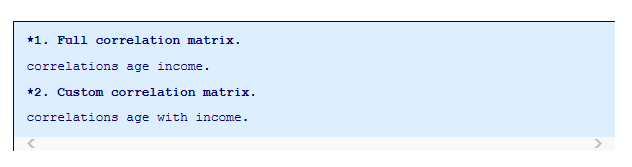## SPSS Correlation Test Output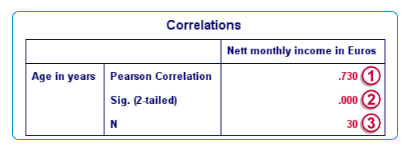The correlation itself, which is 730, indicates a strong (positive) linear relationship between age and income. The values ​​used by Sigs (with 2 sequences) are zero, if the correlation in the population is zero, the chance of finding a correlation in our sample is zero. Hypothesis zero is rejected if p <5, and we conclude that the correlation in this population is not zero (we expect it to be somewhere near 73). More precisely, since this is a two-sequence test, the values ​​include a zero percent chance that the sample correlation is above 73, and another zero chance that is less than -73. The results are based on the population size N = 30, since it is consistent with the sample size, we conclude that there are no missing values ​​in our data.

## Reporting a Correlation Test

When we report a correlation test, the self-correlation of the N value on which it is based is mandatory. Turning to the important test of a reportable rat, it is a strong linear correlation observed between age and income. Pearson coefficient = 73 and p = 00 (2 strains) There are multiple methods of collecting a P value for correlation. With a report similar to what we did, it is not clear which SPSS method was used. Here he used a T test but unfortunately omitted the T value and the amount of freedom. The formulas used by SPSS are under  Help> Algorithms .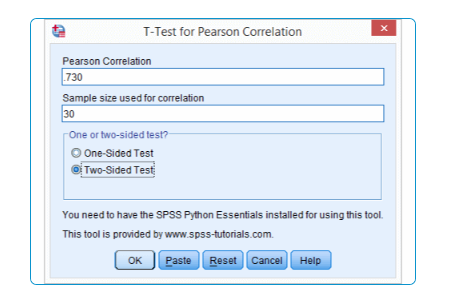In the example for visitors, our data output is printed below: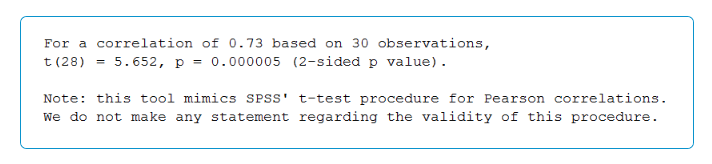Now we have clarified how with our report we have reached the Pearson correlation coefficient of 73; T (28) = 5.7, P = .000 (2 strains)